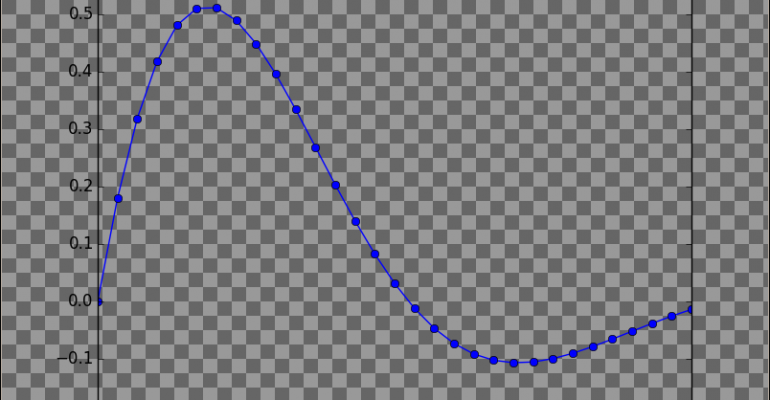Thanks to theidioms.com

### Infosys Tech Scholars Scholarship## Matplotlib Transparent Background – How to save a transparent plot# Matplotlib Transparent Background – How to save a transparent plot

Greetings! Some links on this site are affiliate links. That means that, if you choose to make a purchase, The Click Reader may earn a small commission at no extra cost to you. We greatly appreciate your support!

To save a Matplotlib plot with transparent background, use the `transparent=True` parameter in Matplotlib’s `savefig()` method.

Here is an example of how to save a Matplotlib plot with transparent background:

```import matplotlib.pyplot as plt
import numpy as np

# Sample data points
x = np.linspace(0,6,31)
y = np.exp(-0.5*x) * np.sin(x)

# Plotting
plt.plot(x, y, 'bo-')

# Saving the figure with transparent background
plt.savefig('figure.png', transparent=True)```

This saved plot is now transparent.

This solution has been sourced from the top-voted solution on ‘How to export plots from Matplotlib with transparent background?‘.Do you want to learn Python, Data Science, and Machine Learning while getting certified? Here are some best selling Datacamp courses that we recommend you enroll in:

1. Introduction to Python (Free Course) - 1,000,000+ students already enrolled!
2. Introduction to Data Science  in Python- 400,000+ students already enrolled!
3. Introduction to TensorFlow for Deep Learning with Python - 90,000+ students already enrolled!
4. Data Science and Machine Learning Bootcamp with R - 70,000+ students already enrolled!